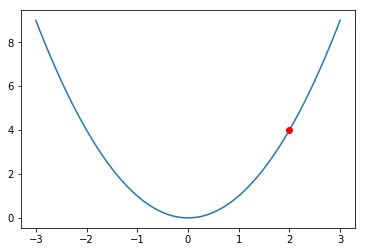# Tensor and Variable

## 把 PyTorch 当做 NumPy 用

PyTorch 的官方介绍是一个拥有强力GPU加速的张量和动态构建网络的库，其主要构件是张量，所以我们可以把 PyTorch 当做 NumPy 来用，PyTorch 的很多操作好 NumPy 都是类似的，但是因为其能够在 GPU 上运行，所以有着比 NumPy 快很多倍的速度。

import torch
import numpy as np

# 创建一个 numpy ndarray
numpy_tensor = np.random.randn(10, 20)


pytorch_tensor1 = torch.Tensor(numpy_tensor)
pytorch_tensor2 = torch.from_numpy(numpy_tensor)


# 如果 pytorch tensor 在 cpu 上
numpy_array = pytorch_tensor1.numpy()

# 如果 pytorch tensor 在 gpu 上
numpy_array = pytorch_tensor1.cpu().numpy()


PyTorch Tensor 使用 GPU 加速

# 第一种方式是定义 cuda 数据类型
dtype = torch.cuda.FloatTensor # 定义默认 GPU 的 数据类型
gpu_tensor = torch.randn(10, 20).type(dtype)

# 第二种方式更简单，推荐使用
gpu_tensor = torch.randn(10, 20).cuda(0) # 将 tensor 放到第一个 GPU 上
gpu_tensor = torch.randn(10, 20).cuda(1) # 将 tensor 放到第二个 GPU 上


cpu_tensor = gpu_tensor.cpu()


# 可以通过下面两种方式得到 tensor 的大小
print(pytorch_tensor1.shape)
print(pytorch_tensor1.size())

torch.Size([10, 20])
torch.Size([10, 20])

# 得到 tensor 的数据类型
print(pytorch_tensor1.type())

torch.FloatTensor

# 得到 tensor 的维度
print(pytorch_tensor1.dim())

2

# 得到 tensor 的所有元素个数
print(pytorch_tensor1.numel())

200


# 答案
x = torch.randn(3, 2)
x = x.type(torch.DoubleTensor)
x_array = x.numpy()
print(x_array.dtype)

float64


## Tensor的操作

Tensor 操作中的 api 和 NumPy 非常相似，如果你熟悉 NumPy 中的操作，那么 tensor 基本是一致的，下面我们来列举其中的一些操作

x = torch.ones(2, 2)
print(x) # 这是一个float tensor

 1  1
1  1
[torch.FloatTensor of size 2x2]

print(x.type())

torch.FloatTensor

# 将其转化为整形
x = x.long()
# x = x.type(torch.LongTensor)
print(x)

 1  1
1  1
[torch.LongTensor of size 2x2]

# 再将其转回 float
x = x.float()
# x = x.type(torch.FloatTensor)
print(x)

 1  1
1  1
[torch.FloatTensor of size 2x2]

x = torch.randn(4, 3)
print(x)

-0.8203 -0.0328  1.8283
-0.1734 -0.1873  0.9818
-1.8368 -2.2450 -0.4410
-0.8005 -2.1132  0.7140
[torch.FloatTensor of size 4x3]

# 沿着行取最大值
max_value, max_idx = torch.max(x, dim=1)

# 每一行的最大值
max_value

 1.8283
0.9818
-0.4410
0.7140
[torch.FloatTensor of size 4]

# 每一行最大值的下标
max_idx

 2
2
2
2
[torch.LongTensor of size 4]

# 沿着行对 x 求和
sum_x = torch.sum(x, dim=1)
print(sum_x)

 0.9751
0.6212
-4.5228
-2.1997
[torch.FloatTensor of size 4]

# 增加维度或者减少维度
print(x.shape)
x = x.unsqueeze(0) # 在第一维增加
print(x.shape)

torch.Size([4, 3])
torch.Size([1, 4, 3])

x = x.unsqueeze(1) # 在第二维增加
print(x.shape)

torch.Size([1, 1, 4, 3])

x = x.squeeze(0) # 减少第一维
print(x.shape)

torch.Size([1, 4, 3])

x = x.squeeze() # 将 tensor 中所有的一维全部都去掉
print(x.shape)

torch.Size([4, 3])

x = torch.randn(3, 4, 5)
print(x.shape)

# 使用permute和transpose进行维度交换
x = x.permute(1, 0, 2) # permute 可以重新排列 tensor 的维度
print(x.shape)

x = x.transpose(0, 2)  # transpose 交换 tensor 中的两个维度
print(x.shape)

torch.Size([3, 4, 5])
torch.Size([4, 3, 5])
torch.Size([5, 3, 4])

# 使用 view 对 tensor 进行 reshape
x = torch.randn(3, 4, 5)
print(x.shape)

x = x.view(-1, 5) # -1 表示任意的大小，5 表示第二维变成 5
print(x.shape)

x = x.view(3, 20) # 重新 reshape 成 (3, 20) 的大小
print(x.shape)

torch.Size([3, 4, 5])
torch.Size([12, 5])
torch.Size([3, 20])

x = torch.randn(3, 4)
y = torch.randn(3, 4)

# 两个 tensor 求和
z = x + y


x = torch.ones(3, 3)
print(x.shape)

# unsqueeze 进行 inplace
x.unsqueeze_(0)
print(x.shape)

# transpose 进行 inplace
x.transpose_(1, 0)
print(x.shape)

torch.Size([3, 3])
torch.Size([1, 3, 3])
torch.Size([3, 1, 3])

x = torch.ones(3, 3)
y = torch.ones(3, 3)
print(x)

print(x)


$$\left[ \begin{matrix} 1 & 1 & 1 & 1 \ 1 & 2 & 2 & 1 \ 1 & 2 & 2 & 1 \ 1 & 1 & 1 & 1 \end{matrix} \right] \ [torch.FloatTensor\ of\ size\ 4x4]$$

# 答案
x = torch.ones(4, 4).float()
x[1:3, 1:3] = 2
print(x)

 1  1  1  1
1  2  2  1
1  2  2  1
1  1  1  1
[torch.FloatTensor of size 4x4]


## Variable

tensor 是 PyTorch 中的完美组件，但是构建神经网络还远远不够，我们需要能够构建计算图的 tensor，这就是 Variable。Variable 是对 tensor 的封装，操作和 tensor 是一样的，但是每个 Variabel都有三个属性，Variable 中的 tensor本身.data，对应 tensor 的梯度.grad以及这个 Variable 是通过什么方式得到的.grad_fn

# 通过下面这种方式导入 Variable

x_tensor = torch.randn(10, 5)
y_tensor = torch.randn(10, 5)

# 将 tensor 变成 Variable
x = Variable(x_tensor, requires_grad=True) # 默认 Variable 是不需要求梯度的，所以我们用这个方式申明需要对其进行求梯度

z = torch.sum(x + y)

print(z.data)

-2.1379
[torch.FloatTensor of size 1]

<SumBackward0 object at 0x10da636a0>


# 求 x 和 y 的梯度
z.backward()


Variable containing:
1     1     1     1     1
1     1     1     1     1
1     1     1     1     1
1     1     1     1     1
1     1     1     1     1
1     1     1     1     1
1     1     1     1     1
1     1     1     1     1
1     1     1     1     1
1     1     1     1     1
[torch.FloatTensor of size 10x5]

Variable containing:
1     1     1     1     1
1     1     1     1     1
1     1     1     1     1
1     1     1     1     1
1     1     1     1     1
1     1     1     1     1
1     1     1     1     1
1     1     1     1     1
1     1     1     1     1
1     1     1     1     1
[torch.FloatTensor of size 10x5]


$y = x^2$的图像如下

import matplotlib.pyplot as plt
x = np.arange(-3, 3.01, 0.1)
y = x ** 2
plt.plot(x, y)
plt.plot(2, 4, 'ro')
plt.show()# 答案
y = x ** 2
y.backward()

Variable containing: